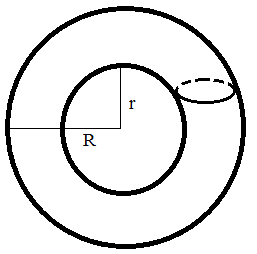# 圆环体积、表面积在线计算器

### App说明$$V = π^2 * (R + r) * (R - r)^2$$

$$S = π^2 * (R^2 - r^2)$$

• R：外半径
• r：内半径

### 使用示例

Comment list ( 0 )